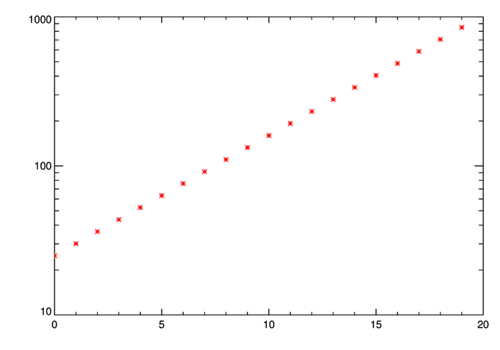Creating an Evenly Spaced Vector in Log Space

QUESTION: Can you show me how to create an evenly spaced vector in log space between a start and ending value? For example, how can I create 20 equally spaced points in logarithmic space between the values 25 and 850.ANSWER: The first step is to find the logarithms of your end points.

s = ALog10(25)
f = ALog10(850)

Next, you create an evenly space vector of exponent values scaled into this data range.

exponents = cgScaleVector(Findgen(20), s, f)

And, finally, you use these exponents to create your actual values.

values = 10.^exponents
cgPlot, values, /YLog, PSym=2, Color='red'

You see the result in the figure below.We created 20 evenly spaced points in log space.

This algorithm has been implemented in the Coyote Library routine cgLogGen.

cgPlot, cgLogGen(20, Start=25, Finish=850), /YLog, PSym='red'